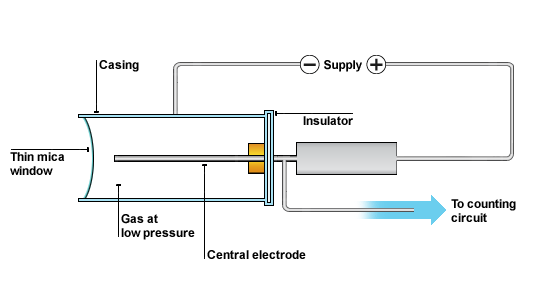# Radiation

## Radiation

Some atomic nuclei are unstable. Notice how we say the nucleus is unstable rather than the atom being unstable. This is an important distinction and will lose you marks if you say atom rather than nucleus.

A nucleus can be unstable for the following reasons:

1. The nucleus has too much energy
2. Too many neutrons
3. Too few neutrons

This instability can cause a nucleus to release radiation in order to become more stable. This radiation originates from the nucleus and nowhere else.

There are four different types of nuclear radiation: Alpha (𝞪), beta (𝞫), gamma (𝞬) and a neutron

An 𝞪 particle is made up of two protons and__ two neutrons__. It is a helium nucleus and therefore has no electrons.

A 𝞫- particl__e is an __electron, originating from the nucleus.

A 𝞫+ particle __is a positron__, originating from the nucleus.

𝞬 radiation is a high energy electromagnetic wave. These have really short wavelengths and high frequencies.

## Background Radiation

Background radiation is radiation that occurs naturally. The main locations for radiation is cosmic rays from space and radioactive rocks and gas in the Earth.

Cosmic rays are emitted from the Sun amongst other stars. Earth’s atmosphere cuts out the majority of the harmful radiation (fortunately).

Radioactive rocks and __gas __occur naturally in the Earth’s crust. Some places have a higher background radiation count due to this. You can easily see where in the UK you have higher amounts of background radiation from the Earth.## Detection

There are a few ways to detect radiation. One of which is a Geiger-Muller tube (GM tube).

The GM tube is a hollow cylinder filled with a gas at low pressure. The tube has a thin window made of mica at one end. There is a central electrode inside the GM tube. A voltage supply is connected across the casing of the tube and the central electrode as shown in the following diagram.When an alpha or beta or gamma radiation enters the tube it produces ions in the gas. The ions created in the gas enable the tube to conduct. A current is produced in the tube for a short time. The current produces a voltage pulse. Each voltage pulse corresponds to one ionising radiation entering the GM tube. The voltage pulse is amplified and counted.

Another way is photographic film. As it sounds, when radiation hits the film, it ionises it and causes the film to change. This is just like photographic film in photography. The film can be developed to show the presence of radiation.

## Properties

𝞪 particles have a range of 3-8cm in air - the shortest of the three.

They cannot penetrate skin or paper and they have the largest __ionisation power of +2 __(this comes from its charge).

𝞫- particles have a range of 1-5m in air - the second furthest.

They cannot penetrate a sheet of aluminium and they have an ionisation power of -1.

𝞫+ particles have a range of 1-5m in air - the second furthest.

They cannot penetrate a sheet of aluminium and they have an ionisation power of +1.

𝞬 radiation can travel __infinitely __as it is just an electromagnetic wave.

It can be stopped by thick lead and it has an ionisation power of 0.

## Decay Equations

There is an equation to show how a nucleus changes when it undergoes alpha decay. Look at the following example:Notice how it looks like a maths equation. The top line reads 208 = 4 + 204 and the bottom line reads 84 = 2 + 82. This is how you will be expected to use this decay equation, by substituting in numbers to ensure the equation is balanced.

There is an equation to show how a nucleus changes when it undergoes beta decay. Look at the following example:Notice how it looks like a maths equation. The top line reads 131 = 131 + 0 and the bottom line reads 53 = 54 - 1. This is how you will be expected to use this decay equation, by substituting in numbers to ensure the equation is balanced.

Notice how the symbol for the beta particle is written as an ‘e’. Sometimes it will be an ‘e’ and sometimes a ‘𝞫’. It does not matter which you use in your exam.

Try these: Write down the radioactive decay equation for each question:

1. Plutonium-239 decays by emitting an alpha particle.
2. Iodine-131 decays by emitting a beta particle.
3. Sodium-24 decays by emitting a beta particle.
4. Uranium-238 decays by alpha particle emission.
5. Carbon-14 decays by beta emission.
6. Potassium-40 decays by beta emission.
7. Bismuth- 213 decays by alpha emission.
8. Americium-241 decays by alpha particle emission.
9. Iridium-192 decays by emitting a beta particle.
10. Polonium-210 decays by emitting an alpha particle.

Answers:Why does nuclear radiation occur?
Your answer should include: nucleus / unstable
Explanation: The nucleus is unstable